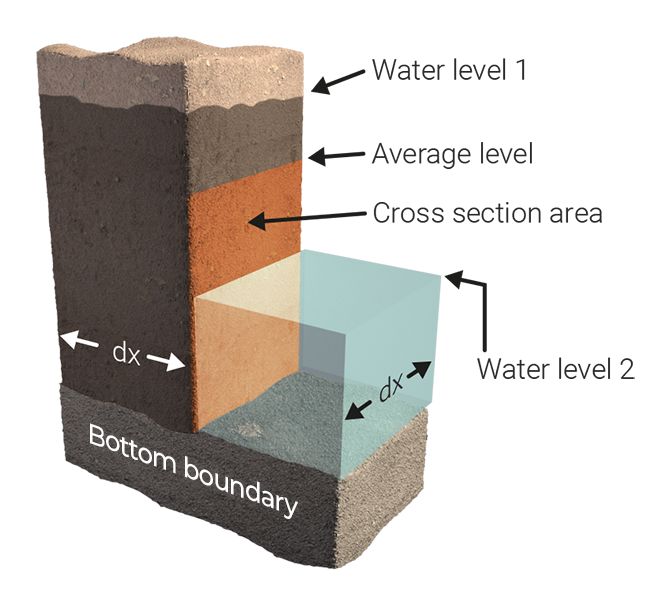# Underground flow formula (Water Overlay)

Underground flow is different from surface flow, since it has to account for the slowdown and porousness of the medium. In general, horizontal underground flow is calculated using formulas described in Harbaugh 2005. However, when an aquifer is present, the aquifer variant is applied.

## Contents

### Default horizontal flowTwo adjacent cells, where underground water level of cell 1 is larger than cell 2.

The flow between the two cells is calculated as:

$\Delta w=w_{1}-w_{2}$$B_{ex}=max(B_{1},B_{2})-D_{ground}$$A_{c}=\Delta x\cdot (w_{avg}-B_{ex})$$C=min(C_{1},C_{2})$$q=\Delta w\cdot C\cdot A_{c}/\Delta x\cdot \Delta t$where:

$w_{n}$= The underground water level of cell n.
$B_{n}$= The surface height of cell n.
$C_{n}$= The ground conductance of cell, defined in GROUND_INFILTRATION_MD of the underground terrain.
$D_{ground}$= The configured ground bottom distance, defined in GROUND_BOTTOM_DISTANCE_M of the Water Overlay.
$A_{c}$= Area of conductance.
$\Delta w$= Underground water level difference.
$\Delta t$= Computational timestep.
$\Delta x$= Size of grid cell.
$w_{avg}$= Averaged underground water level, based on water levels in underground, WATER_STORAGE_PERCENTAGE and potentially the surface water level, when the underground is filled to the top.

### Aquifer formula

When an aquifer is present, its hydraulic diffusivity is used to calculate the water flow.

First the following condition is checked in order to allow water movement through the aquifer:

$V=gw>ah$Based on this condition being true, the transported volume V is calculated:

$V=\Delta gw\cdot KD\cdot \Delta t$Where:

$gw$= Ground water level in the cell;
$ah$= the height of the aquifer at the cell.
$V$= Volume in $m{3}$that flows between the two adjacent cells due to the aquifer.
$\Delta gw$= Ground water level difference between the two adjacent cells;
$\Delta t$= Computational timestep.
$KD$= The AQUIFER_KD attribute of aquifer.

## Related

The following topics are related to this formula.

Features
Aquifer
Formulas
Groundwater level formula
Underground infiltration formula
Models
Underground model
Infiltration model
Substance flow model